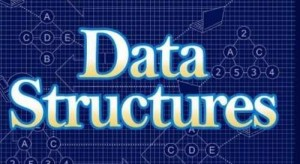21. Mention some of the problem solving strategies?

The most widely strategies are listed below
i)Divide and conquer
ii)Binary doubling strategy
iii)Dynamic programming

22. What is divide and conquer method?

The basic idea is to divide the problem into several sub problems beyond which cannot be further subdivided. Then solve the sub problems efficiently and join then together to get the solution for the main problem.

23. What is the need for the header?
Header of the linked list is the first element in the list and it stores the number of elements in the list. It points to the first data element of the list.

24. Define leaf?
In a directed tree any node which has out degree o is called a terminal node or a leaf.

25. What are the applications of binary tree?

Binary tree is used in data processing.

26. What are the different types of traversing?

The different types of traversing are
i)Pre-order traversal-yields prefix from of expression.
ii)In-order traversal-yields infix form of expression.
iii)Post-order traversal-yields postfix from of expression.

27. Define pre-order traversal?

i)Process the root node
ii)Process the left subtree
iii)Process the right subtree

28. Define post-order traversal?

i)Process the left subtree
ii)Process the right subtree
iii)Process the root node

29. Define in -order traversal?

i)Process the left subtree
ii)Process the root node
iii)Process the right subtree

30. What is meant by sorting?

Ordering the data in an increasing or decreasing fashion according to some relationship among the data item is called sorting.